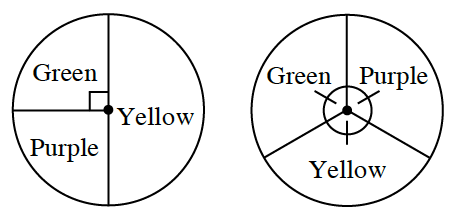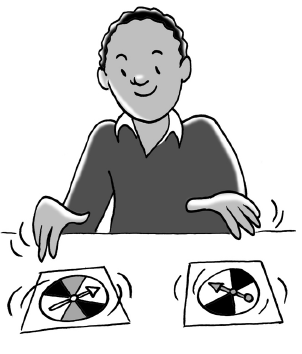### Home > INT2 > Chapter 3 > Lesson 3.1.5 > Problem3-55

3-55.

When he was in first grade, Harvey played games with spinners. One game he especially liked had two spinners and several markers that you moved around a board. You were only allowed to move if your color came up on both spinners.

1. Harvey always chose purple because that was his favorite color. What was the probability that Harvey could move his marker?

Review the Math Notes box in Lesson 3.1.4.

$\frac{1}{12}$

2. Is the probability that Harvey moves his marker calculated from a union or an intersection of events

Refer to the Math Notes box in Lesson 3.1.5.
Is this an "and" or an "or" situation?

3. Was purple the best color choice? Explain.

Does purple have the greatest probability?

4. If both spinners are spun, what is the probability that no one gets to move because the two colors are not the same?

Multiply the probability of the first spinner landing on green with the probability the second spinner will land on purple or yellow.

$\frac{1}{4} \cdot \frac{2}{3} = \frac{1}{6}$

Repeat this step for yellow and purple.

$\frac{1}{6} + \frac{1}{6} + \frac{1}{3} = \frac{2}{3}$

5. There are at least two ways to calculate the answer to part (d). What is another way to solve?